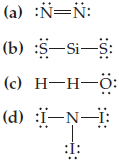×
Get Full Access to Introductory Chemistry - 5 Edition - Chapter 10 - Problem 53p
Get Full Access to Introductory Chemistry - 5 Edition - Chapter 10 - Problem 53p

×

# Answer: Determine what is wrong with each Lewis structure and write the correctISBN: 9780321910295 34

## Solution for problem 53P Chapter 10

Introductory Chemistry | 5th Edition

• Textbook Solutions
• 2901 Step-by-step solutions solved by professors and subject experts
• Get 24/7 help from StudySoup virtual teaching assistantsIntroductory Chemistry | 5th Edition

4 5 1 413 Reviews
23
3
Problem 53P

Determine what is wrong with each Lewis structure and write the correct structure.Step-by-Step Solution:

Solution 53P

Here, we are going to determine what is wrong with the given Lewis structures.

Step 1:

The Lewis dot structure provide a picture of bonding in molecules and ions in terms of the shared pairs of electrons and the octet rule. While such a picture may not explain the bonding and behaviour of a molecule completely, it does help in understanding the formation and properties of a molecule to a large extent. Writing of Lewis dot structures of molecules is, therefore, very useful.

Step 2 of 2

##### ISBN: 9780321910295

Since the solution to 53P from 10 chapter was answered, more than 428 students have viewed the full step-by-step answer. The answer to “?Determine what is wrong with each Lewis structure and write the correct structure.” is broken down into a number of easy to follow steps, and 13 words. Introductory Chemistry was written by and is associated to the ISBN: 9780321910295. This textbook survival guide was created for the textbook: Introductory Chemistry, edition: 5. This full solution covers the following key subjects: structure, correct, lewis, determine, Write. This expansive textbook survival guide covers 19 chapters, and 2046 solutions. The full step-by-step solution to problem: 53P from chapter: 10 was answered by , our top Chemistry solution expert on 05/06/17, 06:45PM.

## Discover and learn what students are asking

Calculus: Early Transcendental Functions : Differential Equations: Growth and Decay
?In Exercises 1-10, solve the differential equation. $$\frac{d y}{d x}=y+3$$

Statistics: Informed Decisions Using Data : Applications of the Normal Distribution
?In Problems 19–22, find the value of ?? ?0.01

Unlock Textbook Solution